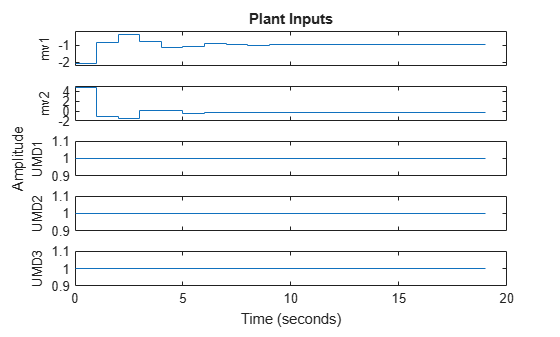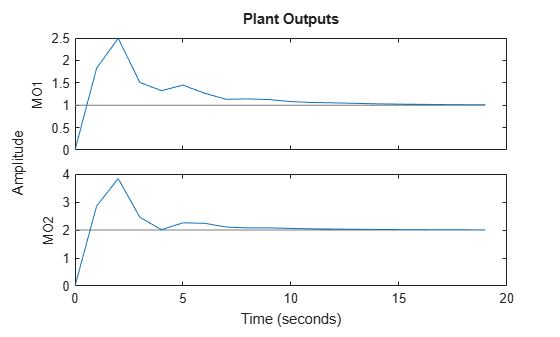# mpcsimopt

MPC simulation options

## Description

When simulating an implicit or explicit MPC controller using the `sim` function, you can specify additional simulation options using an `mpcsimopt` object.

## Creation

### Syntax

``options = mpcsimopt;``

### Description

example

````options = mpcsimopt;` creates a default set of options for specifying additional parameters for simulating an MPC controller with the `sim` function. To specify nondefault values for the properties, use dot notation.```

## Properties

expand all

Simulation plant model initial state, specified as a vector with length equal to the number states in the plant model used for the simulation. To use the default nominal state of the simulation plant model, set `PlantInitialState` to `[]`.

If you do not specify the `Model` option, then the plant model used for the simulation is the internal plant model from the controller. In this case, the default initial controller state is equal to `mpcobj.Model.Nominal.X`.

If you specify the `Model` option, then the plant model used for simulation is `Model.Plant`. In this case, the default initial controller state is equal to `Model.Nominal.X`.

MPC controller initial condition, specified as an `mpcstate` object. Setting `ControllerInitialState = []` is equivalent to setting ```ControllerInitialState = mpcstate(mpcobj)```.

Unmeasured disturbance signal for simulating disturbances occurring at the unmeasured disturbance inputs of the simulation plant model, specified as an array with Nud columns and up to Ntrows, where Nud is the number of unmeasured disturbances, and Nt is the number of simulation steps. If you specify fewer than Nt rows, then the values in the final row of the array are extended to the end of the simulation.

Manipulated variable noise signal for simulating load disturbances occurring at the manipulated variable inputs to the simulation plant model, specified as an array with Nmv columns and up to Ntrows, where Nmv is the number of manipulated variables, and Nt is the number of simulation steps. If you specify fewer than Nt rows, then the values in the final row of the array are extended to the end of the simulation.

Measured output noise signal for simulating disturbances occurring at the measured output of the simulation plant model, specified as an array with Ny columns and up to Ntrows, where Ny is the number of measured outputs, and Nt is the number of simulation steps. If you specify fewer than Nt rows, then the values in the final row of the array are extended to the end of the simulation.

Option to use reference previewing during simulation, specified as one of the following:

• `'off'` — Do not use reference previewing.

• `'on'` — Use reference previewing.

When simulating an explicit MPC controller, you must set `RefLookAhead` to `'off'`.

Option to use measured disturbance previewing during simulation, specified as one of the following:

• `'off'` — Do not use measured disturbance previewing.

• `'on'` — Use measured disturbance previewing.

When simulating an explicit MPC controller, you must set `MDLookAhead` to `'off'`.

Option to enable constraints during simulation, specified as one of the following:

• `'on'` — Use the constraints defined in the controller during simulation.

• `'off'` — Simulate the controller without any constraints.

Plant model to use for simulation, specified as one of the following:

• `[]` — Simulate the controller against its internal plant model (`mpcobj.Model`). In this case, there is no plant-model mismatch.

• LTI system object — Simulate the controller against the specified LTI plant. The specified plant must have the same input and output group configuration as `mpcobj.Model.Plant`. To set this configuration, use `setmpcsignals`.

• Structure with fields `Plant` and `Nominal` — Simulate the controller using the specified plant (`Plant`) and nominal conditions (`Nominal`).

`Model` sets the actual plant (not the internal prediction model of the controller) to be used in closed-loop or open-loop simulations. To test the controller robustness against a plant model mismatch, use this option to specify a plant that differs from the controller internal plant model.

If you do not specify nominal conditions, `Model.Nominal.U` and `Model.Nominal.Y` are inherited from `mpcobj.Model.Nominal`. `Model.Nominal.X` and `Model.Nominal.DX` are only inherited if both plants are state-space objects with the same state dimension.

To specify the initial state of this simulation plant model, use the `PlantInitialState` option.

Option to display the simulation status bar, specified as one of the following:

• `'off'` — Do not display the status bar.

• `'on'` — Display the status bar.

Sequence of manipulated variables to use during open-loop simulation, specified as an array with Nmv columns and up to Ntrows, where Nmv is the number of manipulated variables, and Nt is the number of simulation steps. If you specify fewer than Nt rows, then the values in the final row of the array are extended to the end of the simulation. The specified manipulated variable signals must include any manipulated variable offsets. If `MVSignal` is an empty array (default) then a value of 0 is assumed for all the manipulated variables.

`MVSignal` is ignored if `OpenLoop` is set to `'off'`.

Option to run an open-loop simulation, specified as one of the following:

• `'off'` — Perform a closed-loop simulation.

• `'on'` — Perform an open-loop simulation.

Option to include output noise in measured output plots, specified as one of the following:

• `'off'` — Do not include output noise in plots

• `'on'` — Include output noise in plots

Enable this option to view the output disturbance rejection behavior of the controller during closed-loop simulations.

## Object Functions

 `sim` Simulate an MPC controller in closed loop with a linear plant

## Examples

collapse all

Simulate the MPC control of a multi-input, multi-output (MIMO) system with a mismatch between the predicted and actual plant models. The system has two manipulated variables, two unmeasured disturbances, and two measured outputs.

Define the predicted plant model.

```p1 = tf(1,[1 2 1])*[1 1;0 1]; plantPredict = ss([p1 p1]); plantPredict.InputName = {'mv1','mv2','umd3','umd4'};```

Specify the MPC signal types.

`plantPredict = setmpcsignals(plantPredict,'MV',[1 2],'UD',[3 4]);`

Create the MPC controller.

`mpcobj = mpc(plantPredict,1,40,2);`
```-->The "Weights.ManipulatedVariables" property is empty. Assuming default 0.00000. -->The "Weights.ManipulatedVariablesRate" property is empty. Assuming default 0.10000. -->The "Weights.OutputVariables" property is empty. Assuming default 1.00000. ```

Define the unmeasured input disturbance model used by the controller.

```distModel = eye(2,2)*ss(-0.5,1,1,0); mpcobj.Model.Disturbance = distModel;```

Define an actual plant model which differs from the predicted model and has unforeseen unmeasured disturbance inputs.

```p2 = tf(1.5,[0.1 1 2 1])*[1 1;0 1]; plantActual = ss([p2 p2 tf(1,[1 1])*[0;1]]); plantActual = setmpcsignals(plantActual,'MV',[1 2],'UD',[3 4 5]);```

Configure the unmeasured disturbance and output reference trajectories.

```dist = ones(1,3); refs = [1 2];```

Create and configure a simulation option set.

```options = mpcsimopt(mpcobj); options.UnmeasuredDisturbance = dist; options.Model = plantActual;```

Simulate the system.

`sim(mpcobj,20,refs,options)`
```-->Converting model to discrete time. -->Assuming output disturbance added to measured output channel #1 is integrated white noise. -->Assuming output disturbance added to measured output channel #2 is integrated white noise. -->The "Model.Noise" property is empty. Assuming white noise on each measured output. -->Converting model to discrete time. -->The "PredictionHorizon" property is empty. Assuming default 10. -->The "ControlHorizon" property is empty. Assuming default 2. -->The "Weights.ManipulatedVariables" property is empty. Assuming default 0.00000. -->The "Weights.ManipulatedVariablesRate" property is empty. Assuming default 0.10000. -->The "Weights.OutputVariables" property is empty. Assuming default 1.00000. ```## Version History

Introduced before R2006a